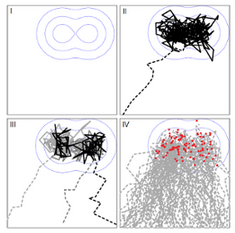#### Mathematical Modeling and Statistical Inference for Biological Systems and Data

Paul-Henry Cournède, Sarah Lemler, Véronique Letort

The « Biomathematics » team is interested in:

• mathematical modeling in biology, whether it is mechanistic modeling of systems or data-driven modeling
• mathematical analysis of models, particularly in the context of dynamic stochastic systems, (stochastic differential equations, hidden Markov models, continuous-time Markov models, graphic models, particle systems in interaction ...) and their simulation
• statistical inference for these models (theoretical analysis of estimators, Monte Carlo methods for Bayesian estimation of state space systems, hierarchical models, sensitivity and uncertainty analysis, identification and design experimental, machine learning)
• system optimization and control (especially in uncertain)

The application areas studied in the team are varied:

• Agro-environmental models and plant growth modelling, which is a research axis developed in the laboratory since 2002 within the Digiplante project
• Neuroscience
• Biological regulatory networks
• Analysis of patint follow-up data or epidemiology.